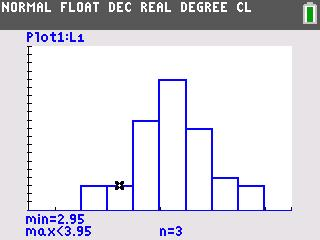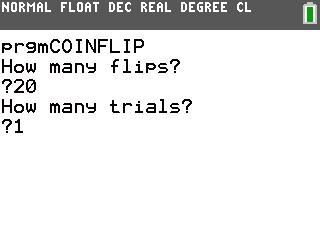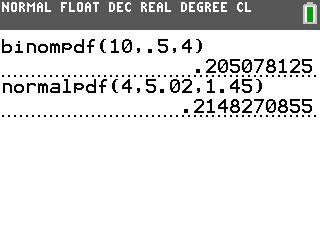# Activities

••• ##### Subject Area

• Math: Algebra II: Data Analysis

• ##### Author9-12

60 Minutes

• ##### Device
• TI-83 Plus Family
• TI-84 Plus
• TI-84 Plus Silver Edition
•TI-84 Plus C Silver Edition
•TI-84 Plus CE
• ##### Software

TI Connect™
TI Connect™ CE

• ##### Accessories

TI Connectivity Cable

• ##### Report an Issue

Modeling Probabilities#### Activity Overview

Students use simulations and graphs to explore what happens when the number of trials of a binomial experiment becomes a large number.

#### Key Steps

•In Problem 1, students simulate a binomial experiment using the program b>COINFLIP. When the simulation runs, each row in list L1 displays the number of heads that occurred for a trial. Students repeat the process using different numbers of trials to determine the shape of the histogram.

•In Problem 2, students explore probability distributions. They simulate 10 flips, for 50 trials and then use a histogram to determine and record the number of times an outcome occurs. Lastly, students will use a formula to calculate the experimental probabilities.

•Students will compare the experimental probabilities with the probabilities calculated with the binomPdf and normPdf commands to determine which distribution best models each situation.